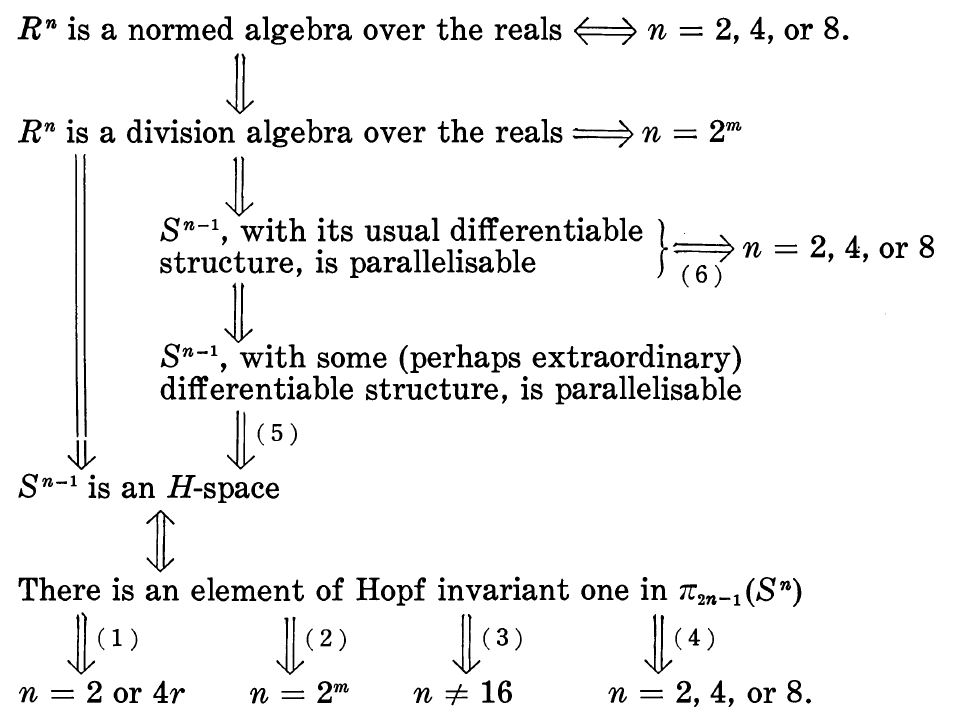Contents

# Contents

## Idea

In algebraic topology, Hopf invariant one refers to homotopy classes of continuous functions between spheres of the form

$\phi \;\colon\; S^{2n-1} \longrightarrow S^n \,,$

hence to elements in the homotopy groups of spheres

$[\phi] \in \pi_{2n-1}(S^n) \,,$

whose Hopf invariant is equal to one:

$h(\phi) = 1 \,.$

Often this is regarded not in the integers but in its quotient to the cyclic group of order 2 $\mathbb{Z}/2$, where it hence says that the actual integer value is an odd number.

The Hopf invariant one problem, once a famous open problem, solved in Adams 60, is the classification of maps for which Hopf invariant is indeed equal to one.

## Properties

### Relation to H-space structure and normed division algebras

The existence of an element of Hopf invariant one in $\pi_{2n-1}(S^n)$ is equivalent to the existence of an H-space structure on $S^{n-1}$.

A celebrated theorem due to (Adams 60, introducing and using the Adams spectral sequence) states that maps of Hopf invariant one correspond precisely to the the Hopf constructions on the four normed division algebras (see also at Hurwitz theorem): the real Hopf fibration, the complex Hopf fibration, the quaternionic Hopf fibration and the octonionic Hopf fibration.## History

Stable homotopy theory emerged as a distinct branch of algebraic topology with Adams‘ introduction of his eponymous spectral sequence and his spectacular conceptual use of the notion of stable phenomena in his solution to the Hopf invariant one problem.

exceptional spinors and real normed division algebras

Lorentzian
spacetime
dimension
$\phantom{AA}$spin groupnormed division algebra$\,\,$ brane scan entry
$3 = 2+1$$Spin(2,1) \simeq SL(2,\mathbb{R})$$\phantom{A}$ $\mathbb{R}$ the real numberssuper 1-brane in 3d
$4 = 3+1$$Spin(3,1) \simeq SL(2, \mathbb{C})$$\phantom{A}$ $\mathbb{C}$ the complex numberssuper 2-brane in 4d
$6 = 5+1$$Spin(5,1) \simeq$ SL(2,H)$\phantom{A}$ $\mathbb{H}$ the quaternionslittle string
$10 = 9+1$Spin(9,1) ${\simeq}$SL(2,O)$\phantom{A}$ $\mathbb{O}$ the octonionsheterotic/type II string

The original proof that the only maps of Hopf invariant one are the Hopf constructions on the four normed division algebras is due to